# How to Calculate and Solve for the Current, Time and Quantity of Charge of an Electrolysis | The Calculator Encyclopedia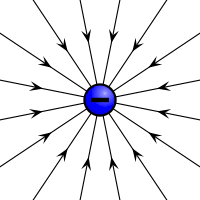The image above represents the quantity of charge.

To compute for the quantity of charge, two essential parameters are needed and these parameters are current (I) and time (T).

The formula for calculating the quantity of charge:

Q = It

Where;
Q = Quantity of charge
I = Current
T = Time

Let’s solve an example;
Find the quantity of charge with a current of 24 and time of 12.

This implies that;
I = Current = 24
T = Time = 12

Q = It
Q = 24 x 12
Q = 288

Therefore, the quantity of charge is 288 Coulombs (C).

Calculating the Current (I) using the Quantity of Charge and Time.

I = Q / t

Where;
I = Current
Q = Quantity of charge
T = Time

Let’s solve an example;
Given that the quantity of charge is 240 with a time of 14. Find the Current?

This implies that;
Q = Quantity of charge = 240
T = Time = 14

I = Q / t
I = 240 / 14
I = 17.14

Therefore, the current is 17.14 ampere.

Calculating the Time (t) when the Quantity of Charge and Current is Given.

t = Q / I

Where;
I = Current
Q = Quantity of charge
T = Time

Let’s solve an example;
Find the time of a quantity of charge of 90 with a current of 30.

This implies that;
I = Current = 30
Q = Quantity of charge = 90

t = Q / I
t = 90 / 30
t = 3

Therefore, the time is 3 secs.

Nickzom Calculator – The Calculator Encyclopedia is capable of calculating the quantity of charge.

To get the answer and workings of the quantity of charge using the Nickzom Calculator – The Calculator Encyclopedia. First, you need to obtain the app.

You can get this app via any of these means:

To get access to the professional version via web, you need to register and subscribe for NGN 1,500 per annum to have utter access to all functionalities.
You can also try the demo version via https://www.nickzom.org/calculator

Once, you have obtained the calculator encyclopedia app, proceed to the Calculator Map, then click on Electrolysis under Physics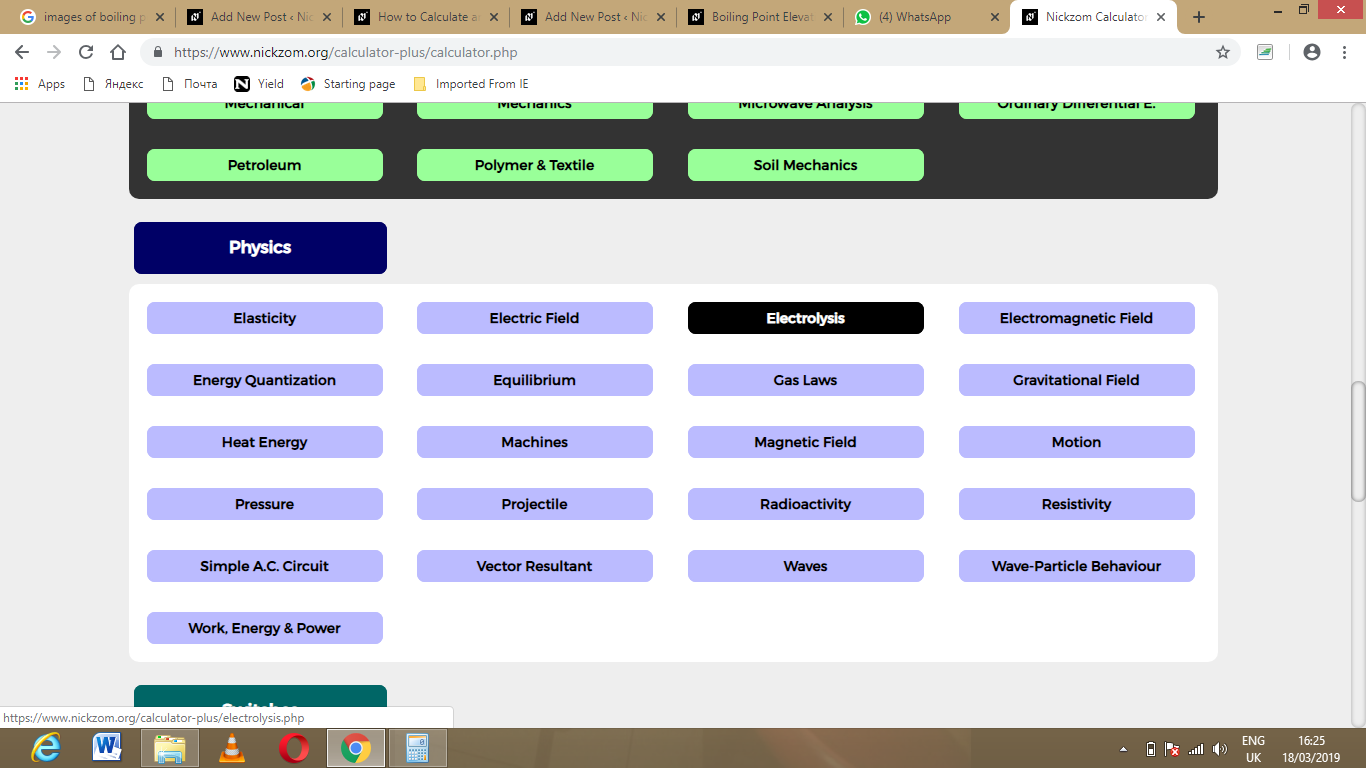Now, Click on Quantity of Charge under Electrolysis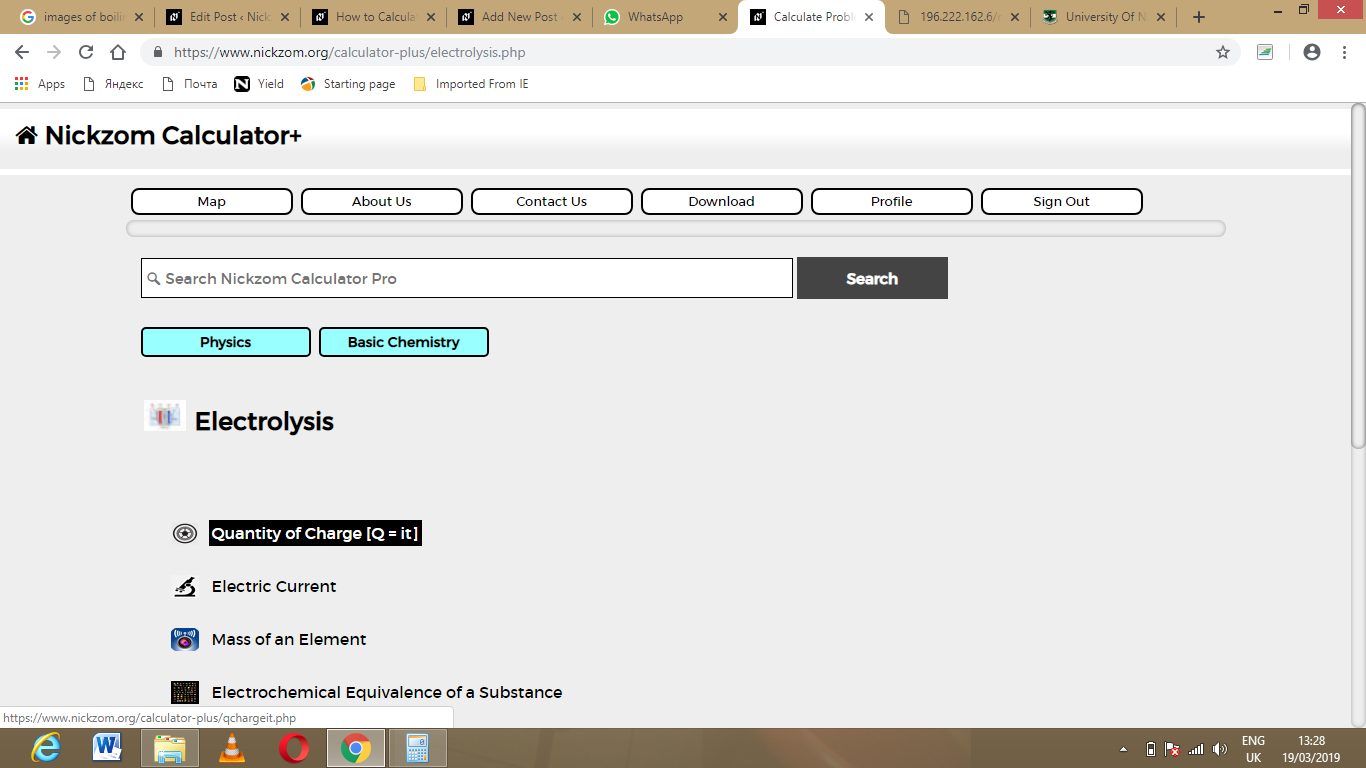The screenshot below displays the page or activity to enter your values, to get the answer for the quantity of charge according to the respective parameters which are the current (I) and time (t).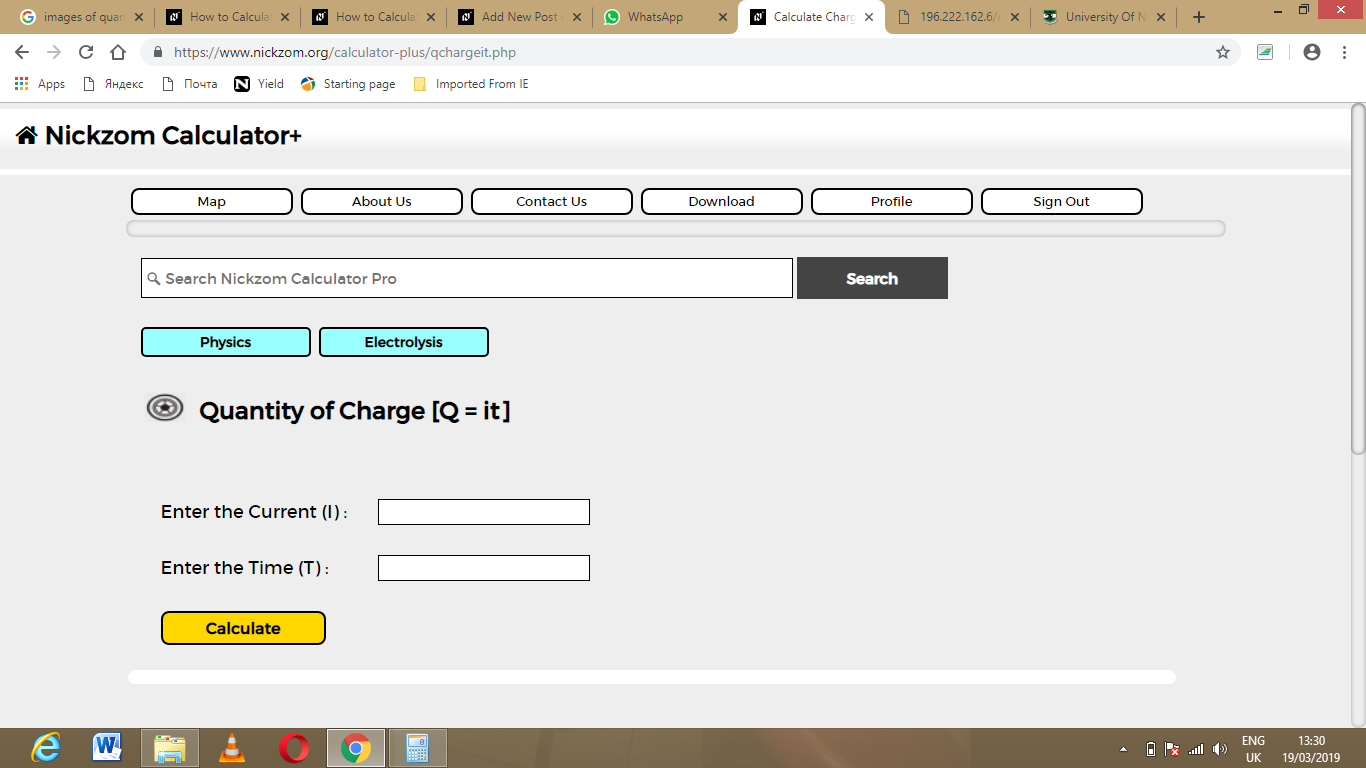Now, enter the values appropriately and accordingly for the parameters as required by the example above where the current (I) is 24 and time (t) is 12.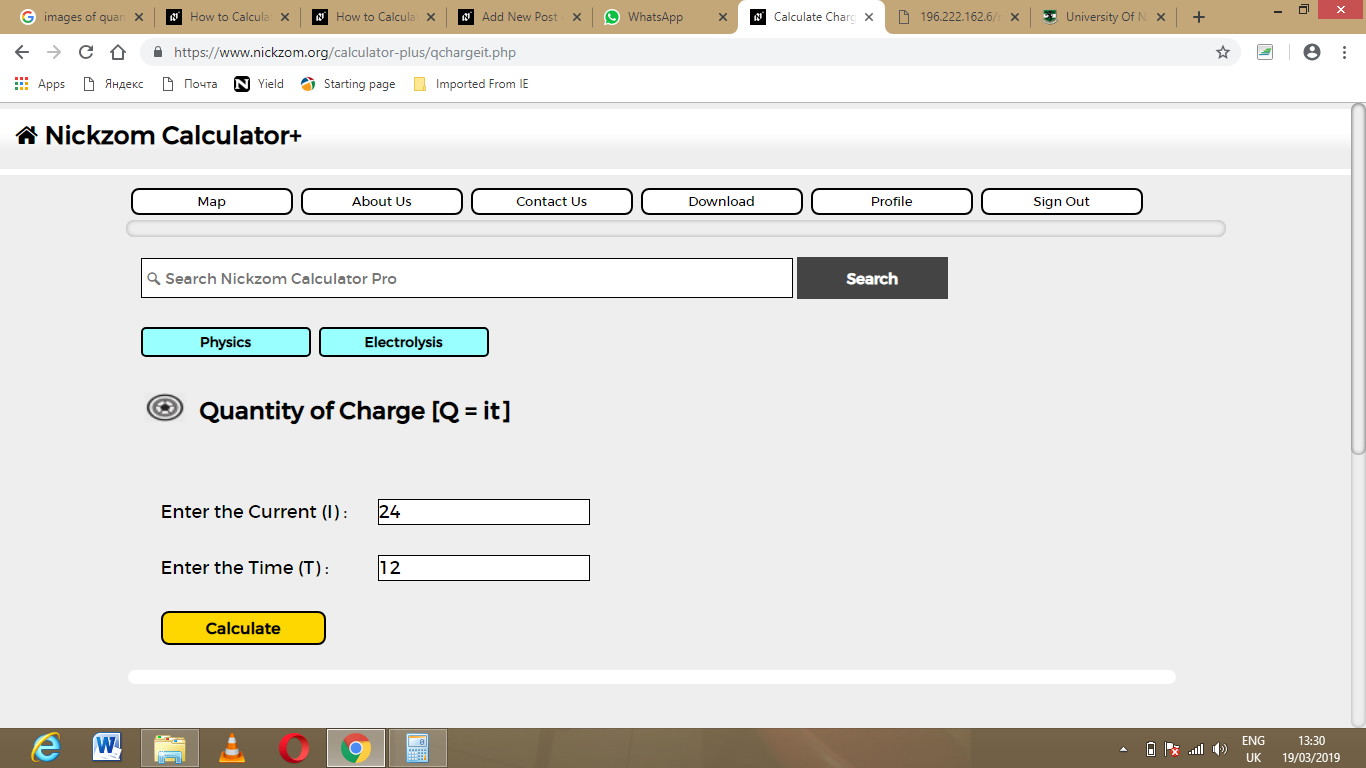Finally, Click on Calculate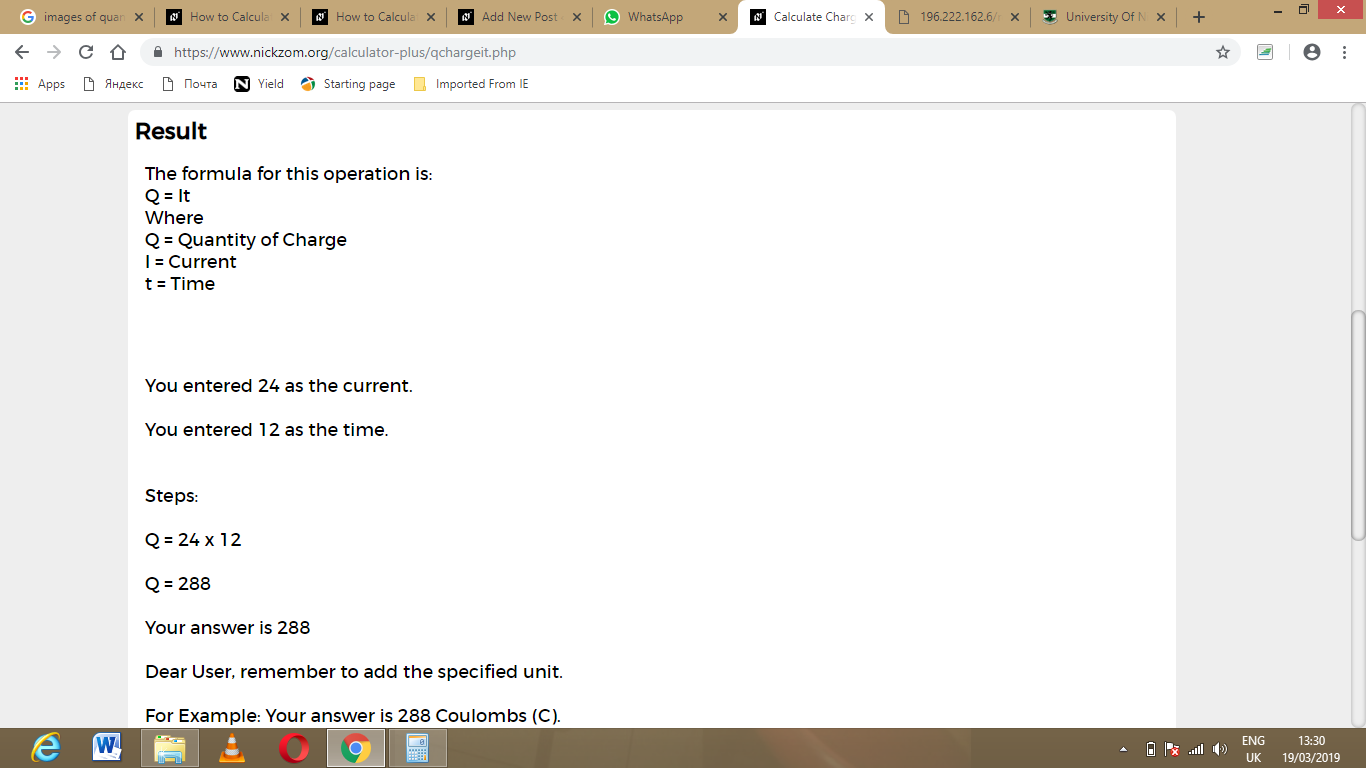As you can see from the screenshot above, Nickzom Calculator – The Calculator Encyclopedia solves for the quantity of charge and presents the formula, workings and steps too.